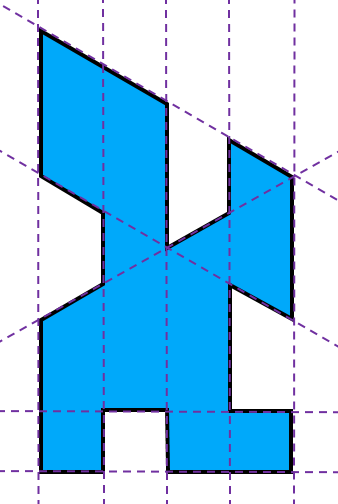# Loyal LinesGiven an $N$-gon, we say that a line is loyal if it intersects the perimeter of the $N$-gon along the entire length of exactly one edge. Determine the largest integer $N$ such that every non-degenerate $N$-gon has a loyal line.

Details and assumptions

• An $N$-gon is non-degenerate if no three consecutive vertices are collinear, or equivalently, that no two consecutive edges are on the same line.
• The above image is an example of a 20-gon that doesn't satisfy the conditions of the problem. The 10 dotted lines are all the lines that contain at least one edge of the 20-gon, however, because all of these lines contain 2 edges, none of them are loyal lines. This shows that the answer is not 20.
×